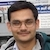# NAEST 2019 Screening Test: Force by the AntsBy

In screening round of National Anveshika Experimental Skills Test ( NAEST 2019 ), a question was asked on the force applied by a group of ants on a food particle. You can see a few ants cooperating with each other to move a food particle.

Question: Assume that the food is slipping on the floor along its length with uniform velocity. The net force exerted by all the ants on the food particle is

1. zero.
2. along the direction of motion.
3. opposite to the direction of motion.
4. at some angle to the direction of motion.

Solution: The food particle is moving in a straight line (along its length) with a uniform velocity. Thus, its acceleration $(a)$ is zero. By Newton's second law ($F=ma$), net force on the food particle is zero.

The food particle is slipping on the floor. Thus, there must be a frictional force on it in a direction opposite to the direction of motion. The forces acting on the particle are

1. force (F) by the ants
2. frictional force (f) opposite to the direction of motion
3. gravitational force (mg) in downwards direction
4. normal reaction (N) from the ground in upwards directionThe net horizontal force on the particle is zero. Thus, force applied by the ants should be equal and opposite to the frictional force. Hence, the net force applied by the ants is along the direction of motion.

The net force F by the ants may be at some angle $\theta$ as shown in the figure. In this case, component of F in the direction of motion balances the frictional force.